# 理解快速排序算法1. 命名左侧序数为 i，初始 i = 1；命名右侧序数为 j，初始 j = len(data)-1（即最后一位）。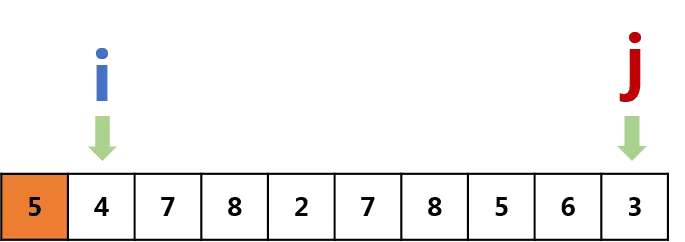2. 让j开始移动并进行判断：

• 若j所在的数字<=5，则让i开始向右移动，直到i所在的数字>5，接着交换data中i, j所对应的数字，即:
```data[i], data[j] = data[j], data[i]
```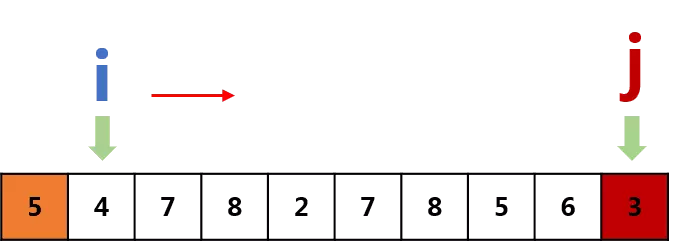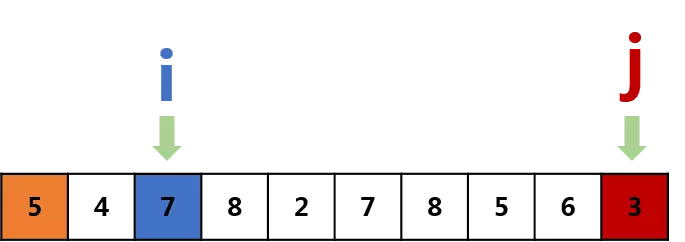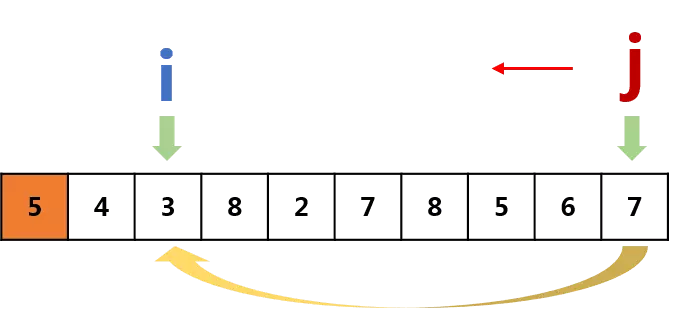• 若j所在数字>5，则忽略，继续向左移动。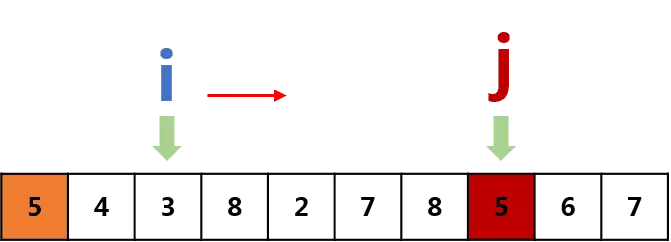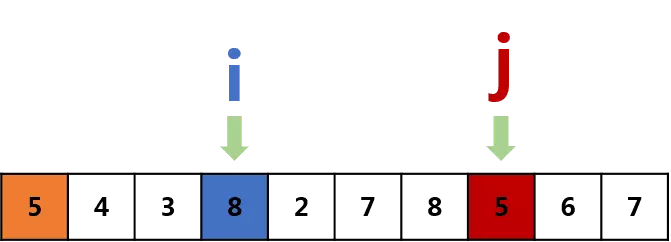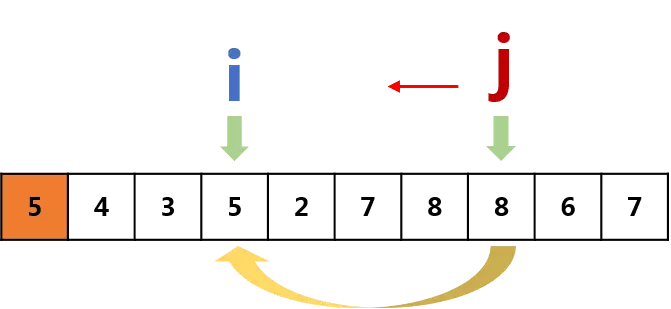3.j == i 时，意味着交换结束，列表除了首位的“标杆数”，其余部分分为<=5和>5两堆，那么我们还应该把“5”放到这两堆中间，让列表看上去更有序。即：

```data, data[j] = date[j], data
```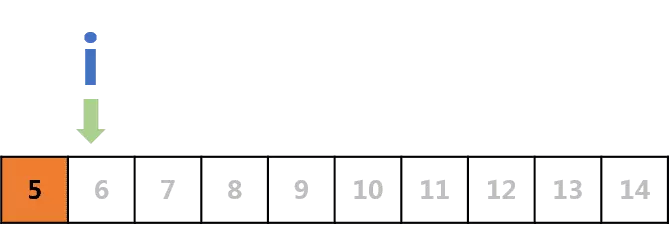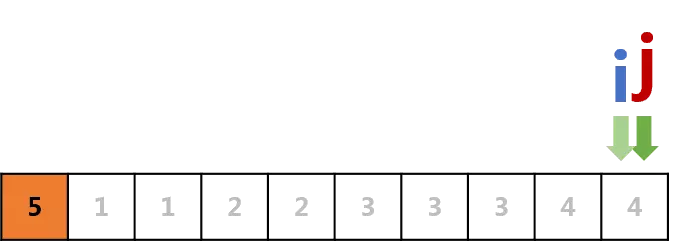```if data[j] <= data:
data, data[j] = data[j], data
```

4. 结束操作，返回此时的data。

```if len(data) == 2:
if data[j] <= data:
data, data[j] = data[j], data
return quicksort(data[left_part]) + quicksort(data[right_part])

if len(data) == 1:
return data
```↓↓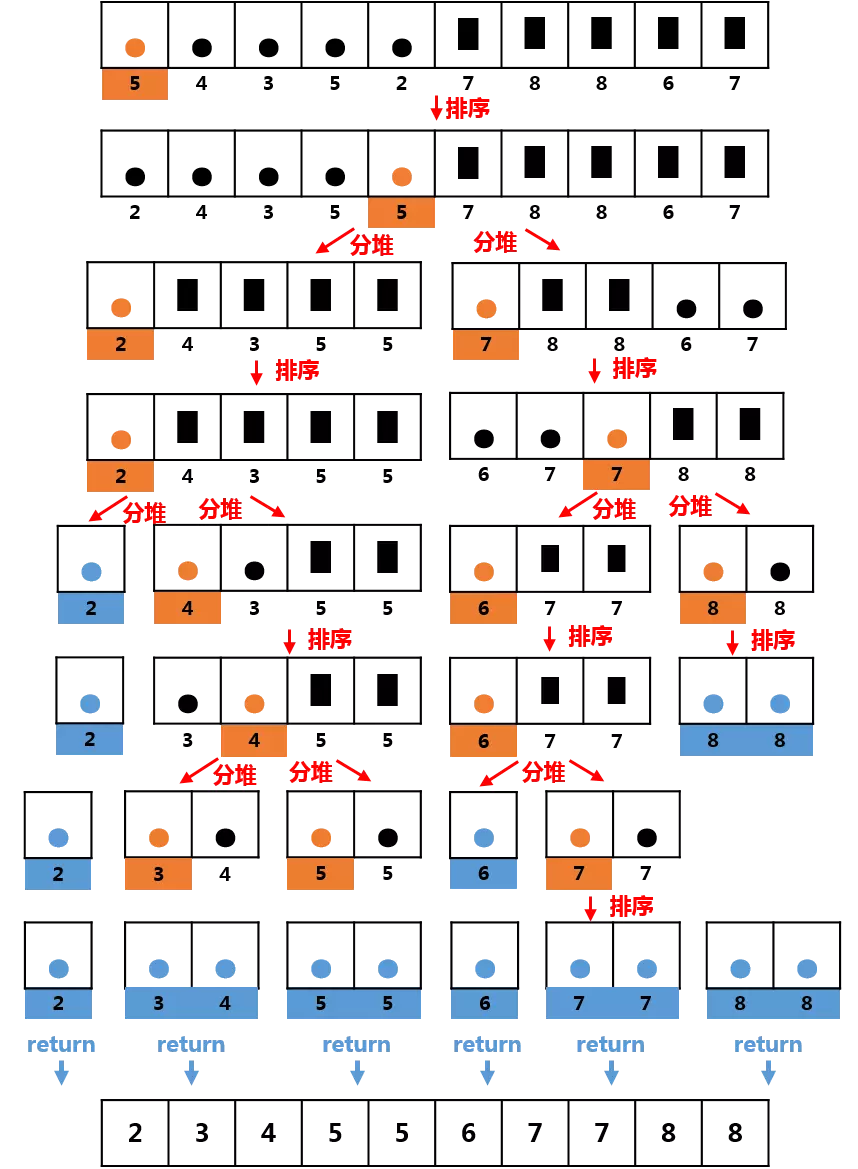```def quicksort(data):     #快速排序
stone = data
i = 1
j = len(data)-1
if len(data) > 1:     #分为len(data) >2和len(data) == 2两种情况，可合并
while j > i:
if data[j] <= stone:
if data[i] > stone:
data[j], data[i] = data[i], data[j]
else:
i += 1
else:
j -= 1
if data[j] <= stone:     #当len(data) == 2时只执行此部分
data, data[j] = data[j], data
return quicksort(data[:j]) + quicksort(data[j:])
else:     #回归条件，len(data) <= 1
return data
```